# 7.4 Radiation diagrams for the rectangular enclosure with a slot on one edge

Radiation diagram shapes vary with the positions, the amplitudes, and the phase relations of the parallel plane excitation currents. For instance, placing a trace symmetric to the symmetry line of the enclosure compensates the first resonance and thus changes the shape of the radiation diagram. The radiation diagrams for the first six resonance modes of a rectangular enclosure with the dimensions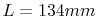,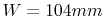,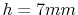and the excitation current position at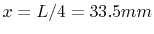,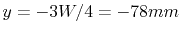are presented in Figure  7.9. After the calculation of the voltages at the slot ports, the diagrams have been obtained with (7.32). A method for an approximate estimation of the enclosure wall influences was presented in . The accurate calculation of the walls requires three-dimensional full wave simulation. However, the directivity and also the wall influence is low for an enclosure with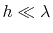and the diagrams obtained with (7.32) provide good first order information about the spherical radiated field distribution.
The diagrams in Figure 7.9 also show significant radiation on the back side of the enclosure. A shift of the source position out of the symmetry line of the enclosure causes asymmetry in the radiation diagram. The main lob is oriented parallel to the symmetry line of the enclosure in the middle of the slot up to the first enclosure resonance. This is also the main lob orientation for many higher order modes.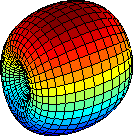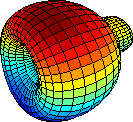(a) (m=1, n=0) (b) (m=2, n=0)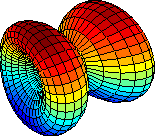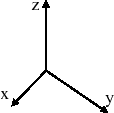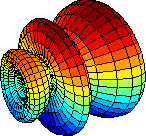(c) (m=2, n=1) (d) (m=3, n=1)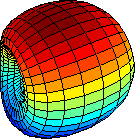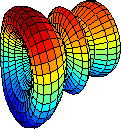(e) (m=1, n=2). (f) (m=3, n=1)
Figure 7.9: Radiation diagrams of a rectangular enclosure with dimensions,and. A single source is driving a current from the cover to the bottom plane at position,.

C. Poschalko: The Simulation of Emission from Printed Circuit Boards under a Metallic Cover